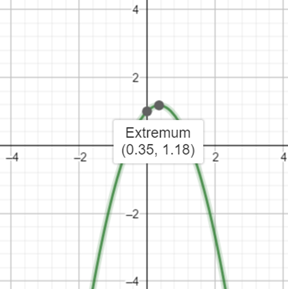# To graph the function using graphing device and find the maximum and minimum value of the function f.### Precalculus: Mathematics for Calcu...

6th Edition
Stewart + 5 others
Publisher: Cengage Learning
ISBN: 9780840068071### Precalculus: Mathematics for Calcu...

6th Edition
Stewart + 5 others
Publisher: Cengage Learning
ISBN: 9780840068071

#### Solutions

Chapter 3.1, Problem 50E

a.

To determine

## To graph the function using graphing device and find the maximum and minimum value of the function f.

Expert Solution

### Explanation of Solution

Given information : Use graphing device to graph the function f(x)=1+x2x2 .

Graph : Sketch the graph using graphing utility.

Step 1: Press WINDOW button to access the Window editor.

Step 2: Press Y= button.

Step 3: Enter the expression f(x)=1+x2x2 which is required to graph.

Step 4: Press GRAPH button to graph the function.

The graph is obtained as:Interpretation :

From the above graph, it can be observed that the maximum value is at (1.18)

b.

To determine

### To Find: The exact maximum or minimum value of the function f(x)=1+x−2x2 and compare it with the above answer.

Expert Solution

The value of maxima is f(122)=(1.18)

both answers up to two decimal places are similar.

### Explanation of Solution

Given: The function is f(x)=1+x2x2

Calculation:

This is a quadratic function with a=2, and b=1 . Thus, the maximum or minimum value occurs at

x=b2a=12(2)=122

Since, a<0 , the function has the maximum value

f(122)=1+(122)2(122)2=1.18

Therefore, both answers up to two decimal places are similar.

### Have a homework question?

Subscribe to bartleby learn! Ask subject matter experts 30 homework questions each month. Plus, you’ll have access to millions of step-by-step textbook answers!